# Pupils

There are 32 pupils in the classroom, and girls are two-thirds more than boys.

a) How many percents are more girls than boys? Round the result to a whole percentage.
b) How many are boys in the class?
c) Find the ratio of boys and girls in the class. Write in the simplest form.

Result

c =  12
d =  20
p =  67 %
f =  3:5

#### Solution:

c+d=32
d = c+ 2/3c

c+d = 32
5c-3d = 0

c = 12
d = 20

Calculated by our linear equations calculator.Leave us a comment of example and its solution (i.e. if it is still somewhat unclear...):Be the first to comment!#### To solve this example are needed these knowledge from mathematics:

Need help calculate sum, simplify or multiply fractions? Try our fraction calculator. Our percentage calculator will help you quickly calculate various typical tasks with percentages. Do you have a system of equations and looking for calculator system of linear equations?

## Next similar examples:

1. College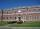At the college are at 57 rooms 194 students. Some rooms are triple and some quad. How many triple and quadruple rooms there is, if it is all fully occupied?
2. Warehouses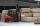To four warehouses is going cement in 25 kg bags. To first one third, to second quarter of the total. The third store got two thirds of the rest, and the last 310 tons came to fourth. How many cement is in all warehouses and how much got every one?
3. Playing Cards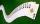Kara has 2 times more cards than Dana, Dana has 4× less than Mary. Together they have 728 cards. How many cards has each of them?
4. Three men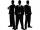Alex is half younger than Jan, which is one-third younger than George. The sum of their ages is 48. How are these three men old?
5. Cups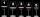We have three cups. In the cups we had fluid and boredom we started to shed. 1 We shed one-third of the fluid from the second glass into the first and third. 2 Then we shed one quarter cup of liquid from the first to the second and to the third. 3 Then
6. Two numbersThe sum of two numbers is 1. Identify this two numbers if you know that the half of first is equal to the third of second number.
7. Forestry workersIn the forest is employed 56 laborers planting trees in nurseries. For 8 hour work day would end job in 37 days. After 16 days, 9 laborers go forth? How many days are needed to complete planting trees in nurseries by others, if they will work 10 hours a d
8. Summerjob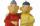Albert, Beny, Cyril and Dan worked on summerjob. From the total revenue Albert received 2/5, Beny 1/6 , 3/10 Cyril and Dan the rest which is 324 euros. How many euros earned every one of them?
9. TV commercials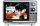For the typical one-hour prime-time television slot, the number of minutes of commercials is 3/8 of the minutes of the actual program. Determine how many minutes of the program are shown in that one hour.
10. Cyclist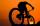Cyclist rode two-fifths of the way the first day. The second day drove 5 km less while traveled three-eighths of route. How many kilometers does he have to finish target?
11. Trees - orchardThe 3/5 trees are apple trees and 1/3 of the trees are cherries. The remaining 5 trees are pear trees. How many trees are in the orchard.
12. Motion2Cyclist started out of town at 19 km/h. After 0.7 hours car started behind him in the same direction and caught up with him for 23 minutes. How fast and how long went car from the city to caught cyclist?
13. Alloy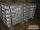The first alloy is a mixture of two metals in the ratio 1:2, the second is a mixture of same metals in the ratio 2:3. At what ratio we have these two alloys put into the furnace to obtain a new metal alloy with ratio 17:27? (All three ratios correspond to.
14. Three shapes1/5 of a circle is shaded. The ratio of area if square to the sum of area of rectangle and that of the circle is 1:2. 60% of the square is shaded and 1/3 of the rectangle is shaded. What is the ratio of the area of circle to that of the rectangle?
15. Lenka and money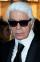When Lenka bought pants from 1/3 of her savings shirt from 1/5 and 1/8 spent at the hairdresser, she is left 328 Kč. How much money was saved? How much hairdresser cost?
16. Ratio of two unknown numbersTwo numbers are given. Their sum is 30. We calculate one-sixth of a larger number and add to both numbers. So we get new numbers whose ratio is 5:7. Which two numbers were given?
17. Two numbersThe sum of the two numbers is 1. Find both numbers if you know that half of the first is equal to one seventh of the second number.